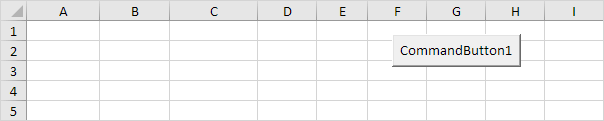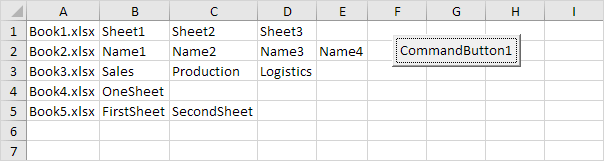# Files in a Directory

Below we will look at a program in Excel VBA that loops through all closed workbooks and worksheets in a directory, and displays all the names.

Download Book1.xlsx, Book2.xlsx, Book3.xlsx, Book4.xlsx and Book5.xlsx and add them to "C:\test\"

Situation:Add the following code lines to the command button:

1. First, we declare two variables of type String, a Worksheet object and two variables of type Integer.

Dim directory As String, fileName As String, sheet As Worksheet, i As Integer, j As Integer

2. To avoid screen flicker, turn off screen updating.

Application.ScreenUpdating = False

3. Initialize the variable directory. We use the Dir function to find the first *.xl?? file stored in this directory.

directory = "c:\test\"
fileName = Dir(directory & "*.xl??")

Note: the Dir function supports the use of multiple character (*) and single character (?) wildcards to search for all different type of Excel files.

4. The variable fileName now holds the name of the first Excel file found in the directory. Add a Do While Loop.

Do While fileName <> ""

Loop

Add the following code lines (at 5, 6, 7, 8 and 9) to the loop.

5. Initialize the variables of type Integer and add the name of the Excel file to the first column of row i.

i = i + 1
j = 2
Cells(i, 1) = fileName

6. There is no simple way to extract data (or sheet names) from closed Excel files. Therefore, we open the Excel file.

Workbooks.Open (directory & fileName)

7. Add all the sheet names of the Excel file to the other columns of row i.

For Each sheet In Workbooks(fileName).Worksheets
Workbooks("files-in-a-directory.xlsm").Worksheets(1).Cells(i, j).Value = sheet.Name
j = j + 1
Next sheet

8. Close the Excel file.

Workbooks(fileName).Close

9. The Dir function is a special function. To get the other Excel files, you can use the Dir function again with no arguments.

fileName = Dir()

Note: when no more file names match, the Dir function returns a zero-length string (""). As a result, Excel VBA will leave the Do While loop.

10. Turn on screen updating again (outside the loop).

Application.ScreenUpdating = True

11. Test the program.

Result: